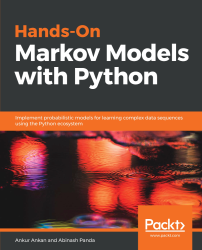•#### Hands-On Markov Models with Python#### Overview of this book

Hidden Markov Model (HMM) is a statistical model based on the Markov chain concept. Hands-On Markov Models with Python helps you get to grips with HMMs and different inference algorithms by working on real-world problems. The hands-on examples explored in the book help you simplify the process flow in machine learning by using Markov model concepts, thereby making it accessible to everyone. Once you’ve covered the basic concepts of Markov chains, you’ll get insights into Markov processes, models, and types with the help of practical examples. After grasping these fundamentals, you’ll move on to learning about the different algorithms used in inferences and applying them in state and parameter inference. In addition to this, you’ll explore the Bayesian approach of inference and learn how to apply it in HMMs. In further chapters, you’ll discover how to use HMMs in time series analysis and natural language processing (NLP) using Python. You’ll also learn to apply HMM to image processing using 2D-HMM to segment images. Finally, you’ll understand how to apply HMM for reinforcement learning (RL) with the help of Q-Learning, and use this technique for single-stock and multi-stock algorithmic trading. By the end of this book, you will have grasped how to build your own Markov and hidden Markov models on complex datasets in order to apply them to projects.
PrefaceFree Chapter
Introduction to the Markov ProcessHidden Markov ModelsState Inference - Predicting the StatesParameter Learning Using Maximum LikelihoodParameter Inference Using the Bayesian ApproachTime Series PredictingNatural Language Processing2D HMM for Image ProcessingMarkov Decision ProcessOther Books You May Enjoy# Dynamic programming

Dynamic programming is a programming paradigm in which we divide a complex problem into smaller sub-problems. We solve these sub-problems and store the results. Whenever we need to recompute the same sub-problem again, we just used our stored results, thus saving us computation time at the expense of using storage space. This technique of caching the results of sub-problems is known as memoization. Therefore, using dynamic programming allows us to speed up our computations by using memoization, and in some cases, it can bring the computational complexity from exponential to linear, as we will see in the following example.

One of the simplest examples of optimization using dynamic programming is computing the nth member of the Fibonacci sequence. Any term in a Fibonacci sequence is the sum of the last two terms, which can be formally defined as follows:

fib...